## The Z mass at a hadron colliderNovember 25, 2008

Posted by dorigo in personal, physics, science.
Tags: , , ,

The Z boson mass has been measured with exquisite precision in the nineties by the LEP experiments ALEPH, OPAL, DELPHI and L3, and by the SLD experiment at SLAC: we know its value to better than a few MeV precision. The PDG gives$M_Z = 91.1876 \pm 0.0021 GeV$. Now, a precise Z mass is an important input to our theory, the Standard Model, and through its measurement, as well as that of other Z-related quantities that the four LEP experiments and SLD measured with great precision, a giant leap forward has been made in the understanding of the subtleties of electroweak interactions.

For an experimental physicist, however, the knowledge of the Z mass is more a tool for calibration purposes than a key to theoretical investigations. Indeed, as I have discussed elsewhere recently, I am working at the calibration of the CMS tracker using the decays of Z bosons, as well as of lower-mass resonances. We take$Z \to \mu \mu$ decays, we measure muon tracks, determine the measured mass of the Z boson with them, and compare the latter to the world average. This provides us with precious information on the calibration of the momentum measurement of muon tracks.

In CMS we will quickly collect large numbers of Z bosons, so statistics is not an issue: we will be able to study the calibration of tracks very effectively with those events. However, when statistics is large, experimentalists start worrying about systematic uncertainties. Indeed, there are several effects that cause a difference between the mass value we reconstruct with muon tracks and the true value of the Z boson mass -the one so well determined which sits in the PDG.

I decided to study one of those effects today: the mass shift due to parton distribution functions (PDF). When you collide protons against other protons, what creates a Z boson is the hard interaction between a quark and an antiquark. These constituents of the projectiles carry a fraction of the total proton momentum, but this fraction -called parton distribution function– is unknown on an event-by event basis. By studying proton collisions in different conditions and environments for a long time, we have been able to extract functions$f_q(x)$ which describe how likely it is that a quark q in the proton carries a fraction x of the proton’s momentum. As an example, if the proton travels at 5 TeV as in LHC, an x value of 0.1 means that the quark q will carry 500 GeV by itself.Now, things are complicated, because each different quark q (u,d,s,c,b) has its own different parton distribution function. The proton contains two valence up-quarks and one valence down-quark: it has a (uud) composition. Those quarks carry a good part of the proton’s momentum, but a large share is due to the rest of partons the proton is made of: sea quark-antiquark pairs, and gluons. Protons do carry antiquarks of all kinds -five in total-, as well as gluons, and these, too, get their own distribution function. A plot of the parton distribution functions of the proton (with a logarithmic x-axis to enhance the low-x behavior) is shown on the right. Note the bumps of u- and d- quark distributions, in blue and green, respectively: those bumps are due to the valence quark contributions.

In reality, things are even more complicated than what I discussed above: you do not simply get away with one function per each of the 11 partons I mentioned thsi far, because these functions have a value which depends on the energy at which you probe the proton,$Q^2$: in a soft collision (which means a small$Q_1$),$f(x, Q^2_1)$ is very different from what it is in a harder one,$f(x, Q^2_2)$ (with a larger$Q_2$).

The reason for the weird behavior of parton distribution functions -their evolution with$Q^2$– is that quarks have the tendency of emitting gluons, becoming less energetic, and this tendency in turn depends on the energy Q at which they are studied. What is stated above is encoded in very famous functions called DGLAP (Dokshitzer-Gribov-Lipatov-Altarelli-Parisi) equations. They are in a sense another consequence of the “asymptotic freedom” exhibited by strongly interacting particles: at high energy they behave as free particles, emitting little color radiation, while at low energy their interaction with the gluon field increases in strength. It is all due to the fact that the coupling constant of the theory,$\alpha_s$, is large at small Q. That constant is not a constant by any means!

You have every reason to be confused now: I was talking about calibrating the CMS tracker using muons, and now we are deep into Quantum ChromoDynamics. What gives ? Well: Z bosons are created by quark-antiquark annihilations, and those are found inside the colliding protons with probabilities which depend on their momentum fraction x, and on the total collision energy Q. Since the PDF of quarks and antiquarks peak at very small values of x, the probability of a collision yielding a Z boson -which has a respectable mass of 91 GeV- is small.  If the Z was lighter, more of them would be produced. Now, the Z boson is a resonance, and like every resonance, it has a finite width. What that means is that not all Z bosons have exactly the same mass: while the peak is at 91.186 GeV, the width is 2.5 GeV, which means that it is not infrequent for a Z boson to have a mass of  89, or 92 GeV, rather than the average value. This is described by the Z lineshape, a function called Breit-Wigner:$F(\Gamma,M) = \frac{\Gamma/2} {(M-M_Z)^2 + \Gamma^2 /4}$.

The function is shown below.As you can see, there is a non-negligible probability that a Z boson has a mass quite different -even a few GeV off- from 91.19 GeV. Now, since Z bosons can be created at masses lower than$M_Z$, they will be privileged by parton distribution functions over masses higher than$M_Z$ by the same amount, because .parton distribution functions are larger at lower x. This creates a bias: the perfectly symmetric Breit-Wigner lineshape gets distorted by the preference of partons to carry a lower fraction of the proton momentum.

The distorsion is very small, but it is very important to take it in account when one wants to use measured Z masses to precisely calibrate the track momentum measurement. To size up the effect of the PDF on the Z lineshape, one can compute an integral of the Breit-Wigner weighted with the PDF$f(x)$, by taking into account the different combinations of quarks which give rise to a Z boson in proton-proton collisions.

A Z can be produced by the following quark-antiquark interactions:

•$u \bar u$: this can originate from a valence u-quark and a sea anti-u-quark, as well as from a sea u-quark and a sea anti-u-quark. The probability that this quark pair creates a Z depends on the coupling of u-quarks to the Z boson, and this probability is a function of some coefficient predicted by electroweak theory. It is proportional to 0.11784.
•$d \bar d$: same as above, but the coupling is proportional to 0.15188.
•$s \bar s$: these can only occur through sea-sea interactions. The coefficient is the same as for d-quarks.
•$c \bar c$: these are due to the small charm component of the proton sea. They get the 0.11784 coefficient as u-quarks too.
•$b \bar b$: these are tiny, but still exist. b-quarks couple to the Z with the 0.15188 factor.
•$t \bar t$: these are basically zero.
•$g g$: gluon-gluon collisions cannot produce a Z boson, because they are vector particles as the Z (spin 1), and a vector-vector-vector vertex is zero by construction. Note that the same does not hold for the Higgs boson, which is a scalar (spin 0) particle: a vector-vector-scalar vertex is possible, and in fact it is the largest contribution to H production at the LHC.Putting everything together, one may compute the shift in the lineshape of the Z, and plot it directly (right, on a logarithmic scale to show the effect on the tails), or as a function of the rapidity of the Z boson, a quantity labeled by the letter Y (the dependence is shown in the last graph of this post, below). Rapidity is a measure of how fast is the Z boson moving in the detector reference frame: when one of the partons has a much larger momentum fraction than the one it is colliding against, the produced Z boson has a large momentum in the direction of the more energetic parton.

The rapidity distribution of Z bosons is shown in the graph below, separately for Zbosons produced by valence-sea collisions (in red) and by sea-sea collisions (in blue).A rapidity Y=0 means that the Z was produced at rest in the detector, +5 is a fast-forward-moving Z, and -5 is a Z moving in the opposite direction with as much speed. As you can see, the valence-sea interactions are the most asymmetric ones, predominantly producing a forward-moving Z boson.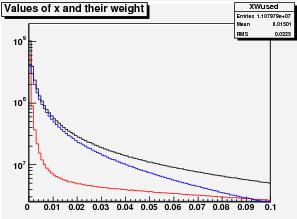On the right here I also plot with the same color-coding the x distribution of quarks taking part in the Z creation. The red distribution has both a very small-x and a very large-x component, highlighting the asymmetric production.

Despite being in black and white, the most interesting plot is however the following one. It shows the average mass of the Z bosons (on the vertical scale, in GeV) as a function of the Z rapidity. The downward shift from 91.186 GeV is relevant -about 0.25 GeV overall- but it increases at large values of rapidity, when one of the two partons has a very small value of x, so that the collision “samples” a rapidly varying PDF for that parton.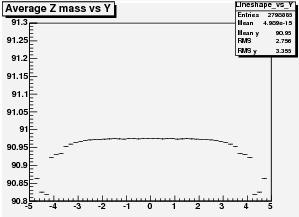The plot on the left here is what is needed as an input for our calibration program: we will have to study how this dependence affects our determination of the momentum scale. A lot of work ahead, but a very enlightening one!

## Upsilon polarization: a surprise from D0August 27, 2008

Posted by dorigo in news, physics, science.
Tags: , , , , , ,

I was surprised by the recent measurement by the D0 collaboration of the Upsilon polarization, which finds a sizable effect which really disagrees with the CDF result, obtained six years ago and based on a 12 times smaller dataset from Run I.

D0 has a large acceptance to muons, and so can detect with good efficiency the$\Upsilon(1S) \to \mu^+ \mu^-$ decays. CDF has a slightly worse acceptance, but its momentum resolution is of a totally different class. Compare the mass distribution of Upsilon mesons published by D0 in their polarization paper, shown below (they refer to different bins of transverse momentum, left to right, and to different fit parametrizations, top to bottom; black points are the data, the red curve is the fit, and the black gaussians are the three Upsilon signals returned by the fit),…with the Run I distribution by CDF on which their old result is based, in the plot below (where the data is the black histogram, and the curve is the fit):Any questions ? Of course, the three Upsilon(1S), (2S), (3S) states merge together in a broad bump in the D0 signal plot, while they stand each on their own in the CDF plot. That’s resolution, baby. Muon momentum resolution is a thing on which Nobel prizes are done and undone.

Despite the lower resolution, D0 can statistically separate the three populations, and measure the Upsilon (1S) and (2S) polarization as a function of the meson transverse momentum. The polarization is defined by the number alpha:$\large \alpha = \frac {\sigma_T-2 \sigma_L} {\sigma_T+2 \sigma_L}$,

where$\sigma_T$ and$\sigma_L$ are the cross-sections for producing a transversely and longitudinally-polarized meson, respectively. The polarization can be measured from the decay angle of the positively-charged muon in the Upsilon rest frame.

D0 had a total of 260,000 Upsilon decays to play with, and they produced a very detailed measurement of the behavior of$\Upsilon(1S)$ and$\Upsilon(2S)$ polarization as a function of the meson$P_T$. This allows a comparison with NRQCD, a factorization approach to the calculation of quarkonium production processes which enshrines in universal non-perturbative color-octet matrix elements the non-computable part, and uses experimental data to fix them.

Confused? Don’t be. Let’s just say that NRQCD is a successful approach at determining several characteristics of charmonium production, and a test of its prediction of the dominance of$\sigma_T$ at high transverse momentum -where gluon fragmentation is the main process for the production of quarkonium in the model- is quite useful.

Another thing to note is that understanding Upsilon production -particularly in the forward region- may be very important for the determination of parton-distribution functions of the proton at very small values of Bjorken x -the fraction of proton momentum carried by a parton. These measurements are very important for the LHC, where interesting physics phenomena will be dominated by very low x collisions.

So let me just jump to the results of the D0 analysis. The polarization plot for the 1S state is shown below. Black points are the D0 measurement, while the green ones show the old CDF result (by the way, it is a shame that CDF does not have a Run II measurement of the Upsilon polarization yet, and you can well say it is partly my fault, since a few years ago I wanted to do this measurement and then had to abandon it…).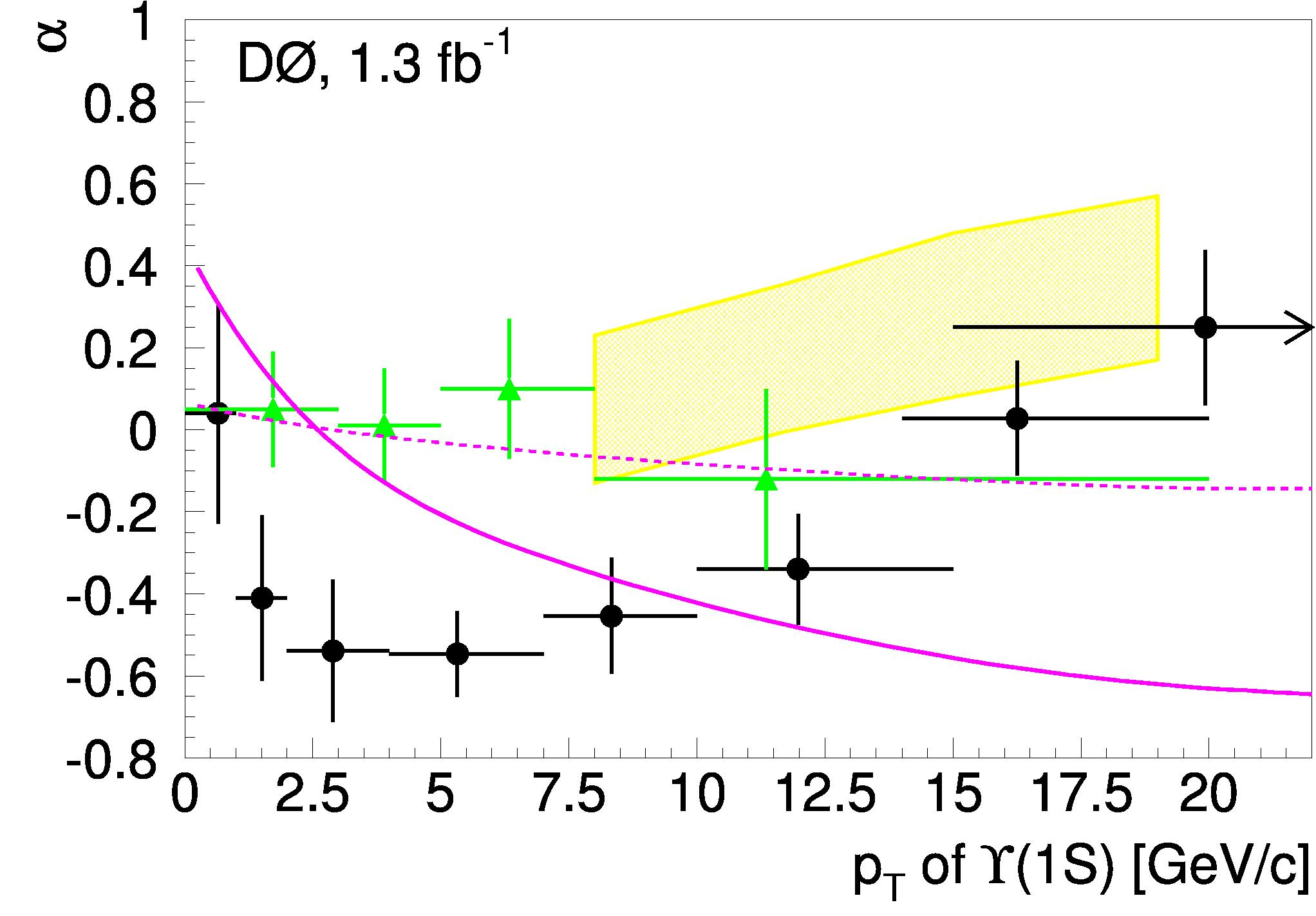As you can see, the D0 result shows a Pt dependence of polarization which is not well matched by the NRQCD predictions (the yellow band, which is only available above 8 GeV of Pt), nor by the two limiting cases of a kt-factorization model. What is worse, however, is that the result comes seriously at odds with the old CDF data points. Who is right and who is wrong ? Or are the two sets of points compatible ?

Well, this is one of those instances when counting standard deviations does not work. The two results have sizable correlated systematic uncertainties among the data points, so moving eight data points up by one sigma collectively may cost much less than$\sqrt{8}$ standard deviations: in the limit that the systematics dominate and they are 100% correlated, moving all points up by$1 \sigma$ just costs one standard deviation… In the case of the D0 points, however, one does not have this information from the paper. One learns that the signal modeling systematics amount to anywhere between 1 and 15%, with the bin with maximum uncertainty being the second one from left; and that background modeling systematics range from 4 and 21%, with the first bin being the worst one. As for the old CDF result, I could not find a detailed description of systematics either, but in that case the precision of the measurement is driven by statistics.

In any case, congratulations to D0 for producing this important new measurement. And I now hope CDF will follow suit with their large dataset of Upsilons too!

## A QCD measurement and why you should care about itAugust 25, 2008

Posted by dorigo in news, physics, science.
Tags: , ,

Quantum ChromoDynamics, the theory of strong interactions, is admittedly not considered the most exciting branch of particle physics at colliders these days. QCD processes make up 99.99% of what happens in hadronic collisions at the Tevatron, or what will happen at the LHC starting this fall: they are usually backgrounds to those much more interesting reactions involving electroweak bosons and leptons, or to the searches for the Higgs boson.

I would like to point out that QCD is in truth a wonderfully complex and beautiful theory, and that QCD measurements are very important. Only by understanding strong interactions in detail can we hope to find new phenomena lying underneath. And don’t even get me started on the need for more studies on low-energy strong interactions -they are really not well understood yet, and in fact precise measurements of strong interaction cross sections are direly needed in cosmology. But let me go back to high-energy high-energy physics.

Today I would like to discuss a precise measurement by CDF which will prove very useful when somebody -me and Mia, for instance- will start studying CMS data in search for the decay$h \to ZZ \to \mu^+ \mu^- b \bar b$: the production of a higgs boson and its subsequent decay into a pair of Z bosons, with a final state including one leptonic Z very easy to identify, and a second one which can be separated from backgrounds by the identification of b-quark jets.

The signal is buried in a large background, namely$Z+ b \bar b$ production where the pair of b-quarks is not coming from a Z boson decay. How large ? Well, we have theoretical calculations, Monte Carlo simulations incorporating them, detector simulations… We have a pretty good idea, but unless we check that these calculations are precise, we are stuck with large systematic uncertainties. One good part of these is due to our limited knowledge of the probability to find a b-quark inside the proton, the b-quark PDF.

A recent result which improves matters has been obtained on the cross section for$Z + b$ production by Andrew Mehta and Beate Heinemann, two very skilled colleagues from Liverpool and Berkeley, respectively. The comparison of the result with theoretical predictions provides a nice confirmation that the latter are in the right ball-park, and an estimate of the level of trust we can put in them. Let me try and describe very briefly how the measurement is produced.

Events with a leptonic Z boson decay are selected from 2.0 inverse femtobarns of proton-antiproton collisions produced by the Tevatron 1.96 TeV synchrotron in the core of the CDF detector. Both$Z \to ee$ and$Z \to \mu \mu$ decays are selected, for a total of about 200,000 events. Among these, the analysis selects those events containing one hadronic jet which has a secondary vertex reconstructed with its charged tracks: the vertex is the signal of the decay of a B-hadron, which contains the long-lived b-quark. By selecting jets with a secondary vertex, their b-purity is increased tremendously.

Below you can see the Z mass peak for events containing a b-quark jet accompanying the dilepton system. The black points are CDF data, the black line is the total of the various contributions, which include, together with the signal, few small backgrounds.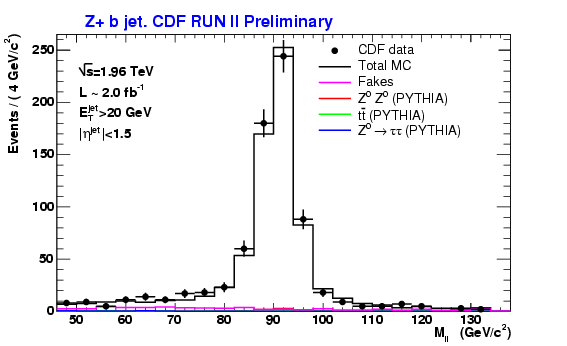To compute a cross-section for$Z + b$ production, there remains one step (ok, I am making things simpler than they really are, for the sake of clarity and space): understanding the fraction of these jets really due to b-quark hadronization. This can be accomplished by studying the invariant mass of all the measured charged tracks originating from the secondary vertex: the mass is larger for real b-quark jets and smaller for charm-quark jets or jets due to lighter quarks or gluons, for which the secondary vertex is due to a random mismeasurement of tracks rather than the true decay of a long-lived particle.Above you can see a fit to the secondary vertex mass distrbution, with the three components. The cyan histogram represents the b-jet fraction, which has a larger vertex mass and amounts for 40% of the total. By measuring the fraction of b-jets one can proceed to measure the cross section, if one knows the efficiency of the selection of Z boson decays and the efficiency of the vertex-finding b-tag algorithm. What I am talking about is the following formula:$\large \sigma_{Zb} = f_b N_{ev} / ( \epsilon_{Z \to ll} \epsilon_{sv} \int L dt )$.

Don’t be scared: the ingredients have all been introduced to you already.$\sigma_{Zb}$ is the cross-section of the process, i.e. the thing that is measured in the analysis.$f_b$ is the fraction of b-jets among those with a secondary vertex, and is extracted by the figure shown above.$\epsilon_{Z \to ll}$ is the fraction of Z bosons which are detected and reconstructed from two observed muons or electrons;$\epsilon_{sv}$ is the efficiency of finding the b-jet with the required energy and with a secondary vertex inside it. Finally,$\int L dt$ is the integrated luminosity of the data used, 2.0/fb.

What is the result ? CDF finds$\sigma_{Zb} = 0.86 \pm 0.14 \pm 0.12 pb$, a small number -eight times smaller than the cross-section for producing a pair of top quarks! Theory calculations at Next-to-Leading-Order (a good level of precision for this calculation) predict$0.53 \pm 0.07 pb$, a figure smaller but not utterly incompatible with the data.

Maybe the most interesting part of the measurement is the ratio between the measured$Zb$ cross section and the cross section for production of one Z boson alone. It is shown in the plot below as a function of the transverse energy of the b-jet, compared to three different Monte Carlo calculations. As you can see, the fraction of Z bosons which are produced together with a b-jet is tiny! The reason has to do with the smallness of the b-quark PDF.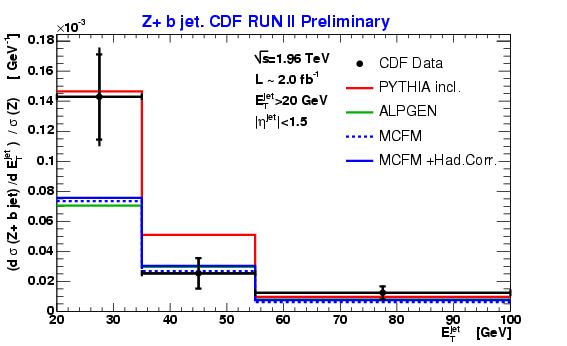## CDF beats theory on the top pair cross sectionAugust 18, 2008

Posted by dorigo in news, physics, science.
Tags: , ,

Among the huge amount of beautiful new measurements produced at the Tevatron by the CDF and D0 experiments last month, just in time for showing at ICHEP 2008, the international conference in High-Energy Physics, there is one which does not make headlines, but it deserves one. It is the measurement of the top pair production cross section, a number which is by itself not terribly informative – it is basically only a check that perturbative calculations with Quantum Chromodynamics work well when they deal with an energy scale where the strong coupling constant is small enough. That is: the above is the only thing one gets from a precise measurement of the top cross section provided one is convinced that there is no other process, so far undiscovered, hiding in top production or top decay.

It is absolutely fair to ask oneself whether top pairs are produced at the Tevatron energy solely by quark-antiquark annihilation and gluon-gluon fusion, the two leading order QCD processes, or whether there is a heavy object X which decays to top quarks, thus enhancing the observed rate of top quarks over what QCD predicts. It is also perfectly legitimate to investigate whether the cross section is in line with predictions regardless of the final state in which one searches for top quarks: some non-standard decays of top could modify the mix. Further, one could hypothesize that the top quark dataset -the data enriched with top events which are used by the experiments to measure cross sections- contains some other process which messes up some of the measurements.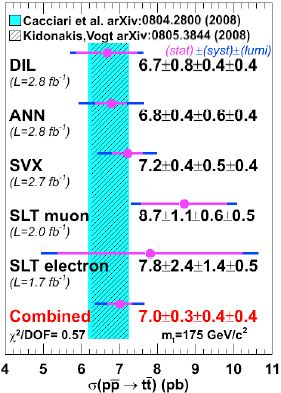The above ideas are to me the most important reason for being interested, 14 years after I first got to know that the top quark existed, in the very precise new determinations of top quark pair cross section obtained by CDF. So let us look at the graph on the right, which details some of the recent determinations, which have been averaged into a result which carries a 8% total uncertainty, beating by 1% the most precise theoretical estimates (9% relative error).

One interesting thing to note is that the cross sections measured with SLT are higher than the average. SLT is the soft-lepton tagging algorithm, which tags b-quark jets coming from top decay through the identification of a muon or an electron embedded in the jet. In Run I, CDF measured a top cross section which was 9 picobarns when using SLT, while about 6 picobarns when using SVX tags -secondary vertices in the jets. Back then, the disagreement was the source of a huge controversy on the hypothesized presence of new physics in the sample of events containing SLT tags. The data did lend itself to some exotic interpretations, but things petered out after years of review and internal diatribas. Now, it does not look like there will be a reprise of that controversy, but the fact remains that SLT cross sections are still there: higher than they should be!

In any case, I salute this new, important result by the CDF top group, and by dozens of dedicated physicists who put their time and efforts into obtaining a very precise measurement. Now the ball is in the theorists’ court, to improve the precision on the theoretical estimate.

UPDATE – ok, a moment after posting the above piece, I looked back at the picture, and I realized that it is not true that the CDF determination is more accurate than theory. It is the theory band which has an 8% uncertainty if I am not mistaken, while CDF has the 9% measurement. That does not change much of the discussion, however, since once the result found by D0 is added to the above one, experiment does get the better hand.

UPDATE II: I also forgot to point interested readers to the public note describing the result!

## The fascinating b quark cross sectionsJuly 10, 2008

Posted by dorigo in news, physics, science.
Tags: , ,

Sometimes I come to think I need a secretary, or even better, a press office. It is such a tough job to keep up to date with the scientific publications popping out on a daily basis, that I sometimes have to completely leave my research aside to foster my own education.

When, however, a new important result escapes my attention for over one year, and the result comes directly from an experiment I am part of, I realize the task is beyond my forces.

Such was indeed the fate of the measurement of correlated b-quark pair production cross section, which CDF published in Phys. Rev. D77, 072004 (2008 ) a couple of months ago (get your preprint here), but which has been around for over a year now. It is especially annoying because it is a very careful measurement which probably settles the issue of b-quark pair cross section, a topic where collider experiments had in the past produced conflicting results. What’s more, the paper is the result of three years of work of a group of friends of mine. Shame on me!

Pairs of b-quarks are produced in hadron collisions by strong interactions (QCD, for Quantum Chromo-Dynamics), typically through the fusion of two gluons. While the production mechanism occurs at an energy high enough to warrant a perturbative calculation -because the strong coupling constant$\alpha_S$ is small enough that it is meaningful to write down the cross section for the hard process as an infinite series of terms in powers of that quantity, the surrounding lower energy phenomena -what happens before the hard scattering, and what happens after it- are non-calculable, and they thus must be evaluated with a fair amount of assumptions.

Before the scattering, you need to “find” in the colliding bodies two suitable partons -mostly gluons, as I said above- of the right energy. The probability to find those partons in the proton and antiproton is a function of their energy, and is described by so-called “parton distribution functions“, which are determined by dedicated experiments.

Similarly non-calculable are the QCD processes responsible for the phase, called “fragmentation” that connects the outgoing b-quarks into a final state with a well-defined observed behavior. The energetic b-quark, leaving the interaction region, extends a color string until the latter “breaks” popping up quark-antiquark pairs which can then bind into color-neutral hadrons – one of them a B-hadron, containing the original b-quark. It is those hadrons which the experiment detects in the tracker and calorimeter system, collectively measuring their energy -or more customarily, their momentum transverse to the beam,$P_T$.

All in all, theoretical calculations of b-quark pair production are a big headache. It is actually surprising that different calculations roughly agree, in fact, once the connection between quark energy and observed B hadron energy is treated with some amount of care. In fact, the comparison of calculations at different level of approximation (at “leading order” or “next-to-leading order” -LO, NLO) present a stable result, which can be therefore trusted to be correct to within 10-20%.

Experimentally, there have been five measurements of the$b \bar b$ production cross section obtained by CDF and D0 in the past. These consider different$P_T$ thresholds for the B hadrons, so to compare them it is meaningful to divide each by the corresponding theoretical prediction. Here are the past results on the experimental/theoretical ratios, based on Run I data:

•$R = 1.2 \pm 0.3$ (CDF, jets with secondary vertex b-tags);
•$R = 1.1 \pm 0.3$ (D0, jets with secondary vertex b-tags);
•$R = 1.5 \pm 0.2$ (CDF, events with one semileptonic muon b-tag jet and the other containing a secondary vertex b-tag);
•$R = 3.0 \pm 0.6$ (CDF, events with two semileptonic muon b-tag jets);
•$R = 2.3 \pm 0.7$ (D0, events with two semileptonic muon b-tag jets).

In total, the average is$R=1.8$ with a 0.8 RMS and a poor overall agreement. Particularly nagging is the dimuon result by CDF, off from unity by more than three standard deviations. With the order-of-magnitude increase of Run II dataset statistics, the matter could and should be straightened!

The new measurement by CDF, based on 740 inverse picobarns of data -roughly eight times more than the former analysis- uses again dimuon events: events where two jets, back-to-back in azimuth, both contain a muon with an impact parameter consistent with the b-quark decay length. The impact parameter is the mismatch between the muon trajectory and the interaction vertex: a large value of$d$ is produced if the muon is not coming from the interaction vertex, but from the decay of a particle that travelled a sizable distance before disintegrating. By fitting the impact parameter distribution of muon tracks in dimuon events, CDF can determine the amount of$b \bar b$ events present in the data sample.Above, the impact parameter, in centimeters, of muon tracks (black points with error bars) is compared to the sum of contributing processes. The $late x b \bar b$ component is shown in cyan. Ignore the blue bars below the main plot – they just show residuals from the fit results.

The measurement is not over once the sample composition of the data is assessed by the above fit, of course: backgrounds have to be shown to be modeled correctly, and the probability that a light hadron is misidentified as a muon must be taken into account. Checks of all kinds can and should be made to ensure a solid result.

As an example: a sizable amount of muons coming from cosmic rays that happened to cross the detector during collider operation need to be removed -they otherwise spoil the impact parameter distribution- by observing the correlation of the impact parameter of the two muons, as shown in the figure below. On the left you can see a population along the diagonal (cosmic muons) – the two muons have the same impact parameter because they are one single track reconstructed as two opposite ones. On the right, data after the requirement that the azimuthal angle between muons of opposite charge is smaller than 3.135 radians are totally devoid of the nasty background.Other checks are made: promptly produced muons can be studied, and tails in their impact parameter distribution sized up, by selecting a sample of Upsilon meson decays. Every time I see a plot of the three resonances (see below) I am reminded of why I love particle physics!So, the analysis is complex, as I said, but we need not delve into the details. So what is the result ? It is found that$R=1.20 \pm 0.21$. The result is not terribly more accurate than the ones quoted above, but the error on R is dominated by the theoretical uncertainty (which is based on a next-to-leading order technique – ok, ignore this detail, and we can both be happy). Therefore, since R should be close to one, or actually exactly one if theory were perfect, can we deduce that the former CDF result ($R=3.0 \pm 0.6$) was plain wrong ? Well, probably yes. In principle, each of the five measurements could be wrong; or all of them, all for the same reason or each for a different one.

The theory predictions could also be the source of deviations from unity in the ratios. What matters, though, is that several of the details with which the present result has been obtained show that the margin for a mistake or a misinterpretation of backgrounds or other instrumental effects, in this case, is really narrow. I have finally -with one year of delay- carefully read the paper, and I am thoroughly convinced that there is no more mystery hiding in the correlated b-quark pair cross section at the Tevatron.

It only remains me to point those of you willing to know more about this measurement to the public page of the analysis, where tens of plots are available together with additional documentation.

## The muon anomaly and the Higgs mass – part IJune 10, 2008

Posted by dorigo in physics, science.
Tags: , ,

Note: despite the technical nature of the matter, I have made an effort to keep this post to a level simple enough that non-scientists should be able to handle. Feedback is welcome!

Nowadays when you are presented with a statement about the inner consistency of the Standard Model of particle physics (SM), and on the range of mass values that a neutral Higgs boson may possess in order to
fit the observed value of several fundamental quantities -all related in a non-trivial way among each other- you are entitled to raise both eyebrows.

Indeed, theorists today speak of the SM as a still-dead entity, because they know it cannot be the ultimate theory. They say it only describes things so well because we have not tested it at energies and forces large
enough. They are quick to point out, if requested (or even without any prodding) that the SM is just “an effective theory”, meaning the opposite (with theorists this happens, at times -it is a lingo barrier rather than backward thinking). They may add that the SM fails to explain the smallness of the mass of the Higgs boson (which has in earnest no apparent reason to be as light as the model wants it), and it does not grant a high-energy unification of fundamental forces in a way which is pleasing to their eye, with the three coupling constants meeting at a single, very large energy scale.

The Standard Model does have those shortcomings. But it has survived more than thirty years of painstaking scrutiny. So your eyebrows have to come down once you realize that, despite all caveats, the predictive power of the combination of existing theory and excellent determination of its free parameters is astonishing. It ain’t no string theory!

There are dozens, but one might say hundreds, of experimental predictions that can be worked out, only to find the SM in exceedingly good health. It is thus not surprising that a handful of these predictions has shown some nagging disagreement with the data in the past. Among them, one might quote a few that are still unresolved today (each of them representing a deviation of measurement from theory by roughly two to three standard deviations): if you accept a list without explanation, I may quote the inconsistency of the measured value of the W boson mass with the observed ratio between neutrino charged-current and neutral-current interactions measured by the NuTeV experiment; the Z boson asymmetry measured by LEP, which shows a difference when measured in leptonic versus hadronic decays; the branching ratio of$Z \to b \bar b$ decays; and the anomalous magnetic moment of the muon.

The anomalous magnetic moment of leptons

A magnetic moment is a property of charged particles with a non-zero value of spin. Although quantum mechanics prevents us from drawing a perfect analogy, a spinning charged sphere develops a magnetic field, and so do charged elementary particles. For them the magnetic moment is easily computed as the product of charge by spin, divided by mass.

The so-called gyromagnetic ratio$g$ is then a pure number defined as the magnetic moment$\mu$ computed in units of its charge divided by twice its mass: for electrons$g_e =\mu / (e/2m_e)$ (where$e$ is the electron charge). The magnitude of$g_e$ describes the magnetic properties of the electron. All charged leptons have gyromagnetic ratios very close to 2, but not quite equal to it. They are exactly 2 in Dirac’s theory of charged fermions, but quantum corrections cause a shift. The deviation of g from 2 -its residual from it- is called anomaly, and it is universally recognized as a very important number,$a_l = (g_l-2)/2$. It is a crucial quantity in electrodynamics, and in particle physics in general, because it is a very small number which can be measured directly, and small non-zero numbers can usually be measured with high accuracy.

Indeed, the electron anomaly$a_e = (g_e-2)/2$ is measured with exquisite precision: it is found that$a_e=1159652180.73\pm28 \times 10^{-12}$, or to within 0.24 parts per billion! It is by its measurement that we know the value of the fine structure constant,$\alpha$ -the fundamental quantity of quantum electrodynamics. Theoretical predictions for$g_e$ can be computed to fractions of a billionth too, so a direct comparison of the two provides a spectacular test of our understanding of the underlying physics.

For muons,$a_\mu$ has been measured with accuracy better than five parts in ten millions, and here theory and measurement differ by 3.4 standard deviations. A paper by Massimo Passera and collaborators, which I will describe in detail tomorrow, discusses the discrepancy and critically analyzes it in terms of the consistency of electroweak fits and low-energy measurements used as input. What I want to do today is to give some preliminary information about the problem, so that I have a chance of explaining to outsiders the details tomorrow.

Calculating the muon anomalySo, what is it exactly that goes in the calculation of the muon anomaly? Well, it boils down to adding together the contributions of different processes which modify the Dirac picture of a muon (whose momentum is labeled “p” and then “p'”) emitting a photon, as in the graph on the right. At “leading order” -that is, when ignoring everything else but the bare-bone electromagnetic process of photon emission- the gyromagnetic ratio is 2 and the anomaly is zero. However, in subatomic physics every process that is not forbidden will happen, with a certain probability which is the square of the “amplitude” of the corresponding particle diagram. Looking beyond the bare-bone process, at “higher order” one needs to consider a huge number of other processes, such as the emission of a second photon by the muon line, with the former subsequently reabsorbed by the muon after the emission of the main outgoing photon, as in the top left graph of the figure below.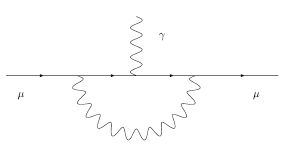As the number of allowed vertices increases, there may be two photon emissions, and fancier things may start happening, as shown below: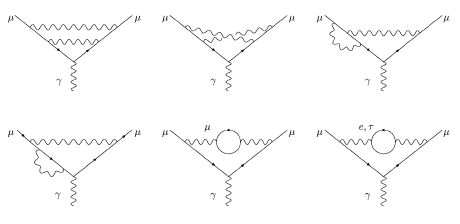Here we have to count at the same order (because they have the same number of vertices -points where three lines meet) diagrams where a single photon is emitted and reabsorbed, but the photon spends some time in the form of a virtual loop of charged leptons, as in the two graphs shown in the lower right above. At still higher orders the diagrams to consider are many more, but they respect the general structure with lines and blobs like those shown in the figures above.

Similarly, we can imagine that the incoming muon emits and reabsorbs a virtual Z boson; or the muon may emit a W boson and temporarily turn into a muon neutrino, as in the diagram in the center in the figure below. It is only by computing each and every possible virtual diagram, with all known particle interactions, that the total quantity we have to compute -the muon anomaly- comes out right.Of course, the number of diagrams diverges as the number of “vertices” (points where particle lines intersect) increases. But physicists are good at performing approximations: by organizing the “higher order” corrections in series of the number of vertices, they can prove that each additional term in the series is a small correction to the former. So they just continue calculating more and more complex diagrams until they have to give up (since the number of diagrams to be computed grows factorially with the number of vertices), and their final result will be good to within the estimated contribution of the first neglected term in the series.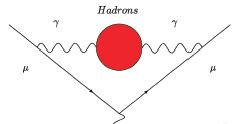From the “nuts and bolts” description I gave above you have by now realized that in the “master” diagram we considered -photon emission from a charged muon line-, strong and electroweak physics enter by necessity at higher order in the perturbation series. Thanks to their electrical charge, even quarks may be produced by a photon fluctuation, and quarks are subject to strong interactions: what they may do, while they are alive in the red blob shown in the graph on the right, needs to concern us. The strength of those interactions will affect the final result for the muon anomaly despite the virtual nature of the quarks! The same goes with W and Z bosons which a muon line can emit (W bosons also connect to photon lines, thanks to their electric charge).

Thus,in the calculation of higher orders of the muon anomaly, there enter not only electrodynamics (which we claim to know inside-out), but also weak and strong interactions: the former are those mediated by the exchange of weak vector bosons (W and Z), the latter are instead those governing the dynamics of quarks -the constituents of nuclear matter- and gluons, the carrier of strong force.

The weakness of an interaction means that as we go to higher orders in the perturbation series diagrams with more vertices become very improbable, and the corrections they cause become small very quickly: the
series converges, and we can calculate it [Post-scriptum: The series does not actually converge – this is a mistake I prefer to not correct, see the comments thread below- but the calculation does work for quantum electrodynamics!]. But for quantum chromodynamics -QCD, the theory of strong interactions- this unfortunately does not happen! Alas, the basic QCD processes we need to compute are
“non perturbative”: higher-order contributions are large and cannot be neglected, no matter how you reorganize your perturbation series.

QCD is a wonderful theory, and high-energy processes can be computed with it with great precision, because at high energy the strong coupling constant (a number by which the probability of any QCD process has to be multiplied once for every particle vertex in the diagram describing the process) is small; but at low energy$\alpha_s$ is large, and perturbation series diverge.

Because of that nasty property of QCD, a calculation of the muon anomaly needs to rely on approximations, modeling, and the knowledge of low-energy QCD. Some processes that help derive the quantities which are used in these approximations are those we measure in low-energy electron-positron scattering experiments: the cross section of these reactions determine how strong an impact some QCD virtual diagrams have in the muon g-2 calculation.

Ok, I think I have dumped above the preliminary information one needs to have in order to read the next, I hope enlightening, post, which will discuss the recent analysis by Massimo Passera and collaborators. In their paper they explain how the upper theoretical bound on the mass of the Higgs boson depends on the amount of uncertainty in low-energy hadronic cross sections one is willing to allow. Those who can’t wait for the post, and can read a hep-ph paper without assistance, are encouraged to get it here.

UPDATE: This link will bring you to the second part of this post.